# Glencoe Geometry Chapter 10: Circles Chapter Exam

Exam Instructions:

Choose your answers to the questions and click 'Next' to see the next set of questions. You can skip questions if you would like and come back to them later with the yellow "Go To First Skipped Question" button. When you have completed the practice exam, a green submit button will appear. Click it to see your results. Good luck!

### Page 1

#### Question 1 1. If the measure of central angle AOB is 62 degrees, what is the measure of inscribed angle ACB?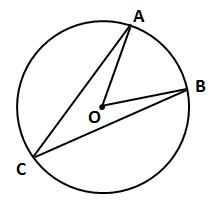#### Question 2 2. In the picture below, if AC is 14 and AB is 10, what is the length of AD?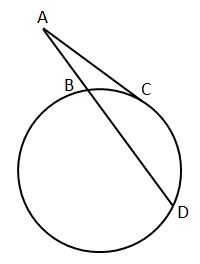### Page 2

#### Question 6 6. In the picture below, if AE is 10, AB is 14 and ED is 16, what is the length of CE?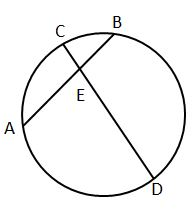#### Question 7 7. Find the center and radius of the following equation: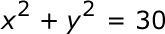#### Question 8 8. In the picture below, if arc CE is 172 degrees and arc BD is 80 degrees, what is the measure of angle CAE?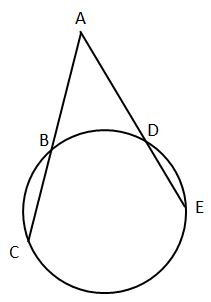#### Question 9 9. In the picture below, if AB is 12, AC is 24 and AD is 10, what is the length of AE?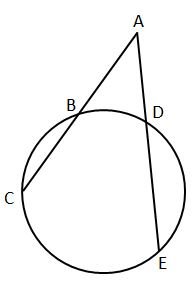### Page 3

#### Question 13 13. In the picture below, if arc AC is 146 degrees and arc BD is 32 degrees, what is the measure of angle AEC?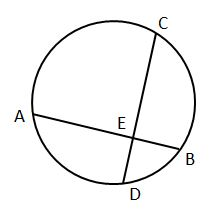### Page 4

#### Question 16 16. The red segment pictured on the circle represents: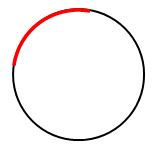#### Question 17 17. In the picture below, if major arc BC is 258 degrees and minor arc BC is 102 degrees, what is the measure of angle BAC?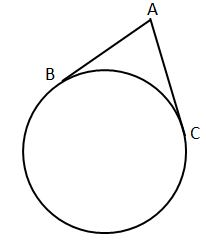#### Question 20 20. Find the center and radius of the following equation: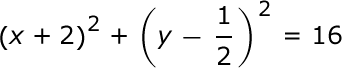### Page 5

#### Question 21 21. If arc AB is 72 degrees, what is the measure of angle ACB?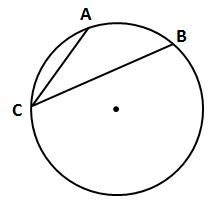#### Question 22 22. Which line is a tangent to the pictured circle?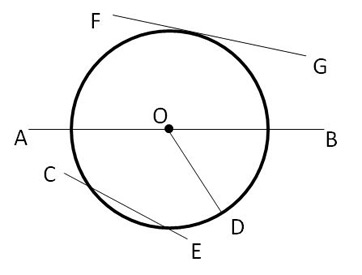#### Question 23 23. What is the area of the shaded area, rounded to the nearest whole number?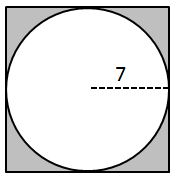#### Question 24 24. What is the area of the shaded area, rounded to the nearest whole number?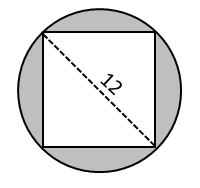#### Question 25 25. Choose the equation for the following circle: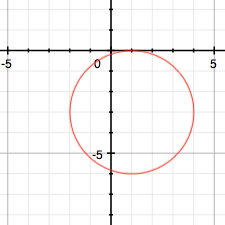### Page 6

#### Question 27 27. In the picture below, if AE is 8, CE is 12 and ED is 5, what is the length of EB?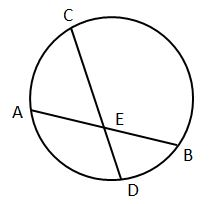#### Question 28 28. If the area of the square is 64, what is the area of the circle, rounded to the nearest whole number?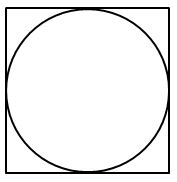#### Question 29 29. Find the center and radius of the following equation: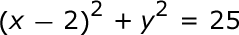#### Question 30 30. If AB and CB are tangent segments, what is the length of CB?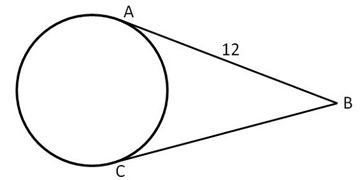#### Glencoe Geometry Chapter 10: Circles Chapter Exam Instructions

Choose your answers to the questions and click 'Next' to see the next set of questions. You can skip questions if you would like and come back to them later with the yellow "Go To First Skipped Question" button. When you have completed the practice exam, a green submit button will appear. Click it to see your results. Good luck!

Support